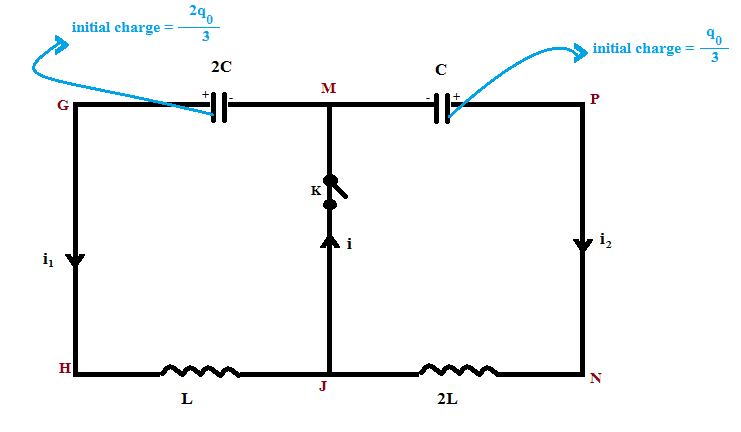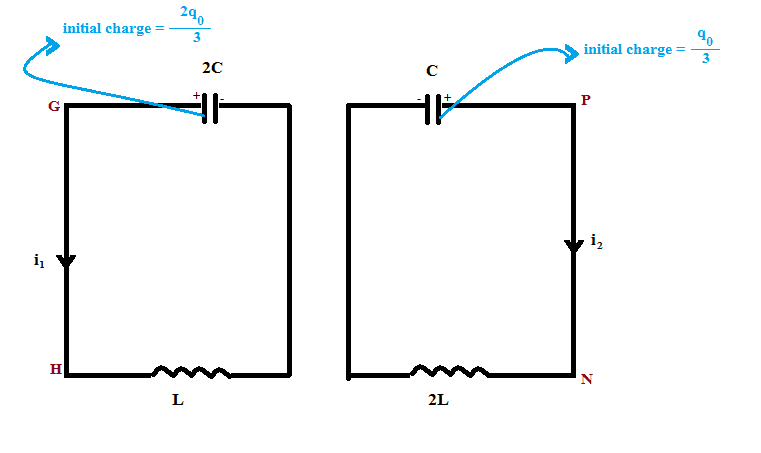# LC circuit

## Homework Statement[/B]
$C$ and $2C$ represent the capacitance of the respective capacitors. $L$ and $2L$ represent the inductance of the respective inductors. Let, the charge on the capacitors GM and PM be $q_1$ and $q_2$ which are variable. $t$ represents time. Initially (when the switch k was closed and $t = 0$) , $q_1 = \frac{2q_0}{3} ; q_2 = \frac{q_0}{3}$. Would it be,
$i_1 = - \frac{dq_1}{dt} ; i_2 = - \frac{dq_2}{dt}$ ?

## Homework Equations

That is conceptual question. No equation is needed.

## The Attempt at a Solution

The actual homework was different. But I need to clear my concept before solving the actual problem. My confusion is, the MGHJ segment (which has the current $i_1$) is connected with both the capacitors. So, won't both the capacitor affect the current $i_1$ ?

gneill
Mentor
The short circuit between M and J when the switch is closed effectively creates two isolated loops as no potential can develop across the short. In other words, M and J become a single node. No current from either loop can stray into the other loop past that short circuit.

•arpon
The short circuit between M and J when the switch is closed effectively creates two isolated loops as no potential can develop across the short. In other words, M and J become a single node. No current from either loop can stray into the other loop past that short circuit.
So, if I change the circuit into the following , I think $i_1$ and $i_2$ won't change. Am I right?gneill
Mentor
Right.

•arpon